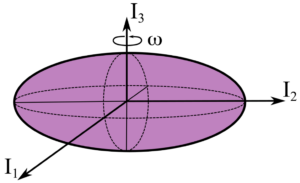# Shifting our View on Neutron Stars with Gravitational Wave Parallax

Authors: Magdalena Sieniawska, David Ian Jones, Andrew Lawrence Miller

First Author’s Institution: Centre for Cosmology, Particle Physics and Phenomenology (CP3), Université catholique de Louvain, Chemin du Cyclotron 2, B-1348 Louvain-la-Neuve, Belgium

Status: To be submitted to MNRAS (closed access)

Astronomers have long used parallax measurements to determine the distance to celestial objects, most notably with the Gaia space telescope. Today’s paper proposes using a similar method to measure the distances to neutron stars with gravitational wave parallax.

### Continuous Gravitational Waves

All of our gravitational wave detections so far have been compact binary coalescence, which is the inspiral and collision of two incredibly dense objects (which may be neutron stars and/or black holes). But, this isn’t the only process that can produce gravitational waves. Spinning compact objects themselves can produce continuous gravitational waves (CGWs), which are detectable over large timescales rather than as a single cataclysmic event.

It is important to note that a perfectly spherical neutron star’s rotation will not produce CGWs. Only a neutron star with long-lasting asymmetry (asymmetry that is misaligned from its rotation axis, at that) will produce CGWs. These can be caused by elastic and/or magnetic deformations or “mountains” on a neutron star’s surface. An example morphology is shown in Figure 1 below.Figure 1: Simple model of a possible asymmetric neutron star shape. The star is elongated along the$I_2$ axis, which is misaligned from the$I_3$ rotation axis, which means that its rotation will produce continuous gravitational waves. (Image credit: Sieniawska & Bejger, 2019)

### A Pair of Parallaxes

Geometric parallax is calculated by measuring the extremely small change in a star’s apparent position on the sky when Earth moves to the opposite side of the Sun in its orbit. With that angle (which is 1 AU divided by the distance) and our knowledge of Earth’s orbital distance, we can use trigonometry to calculate how far away the star is. Gravitational wave parallax follows a similar idea, though the execution is more difficult.

With gravitational wave detectors, it’s not so easy to just take a picture and find a precise location. However, gravitational waves will appear to change slightly in frequency due to the Doppler effect as Earth orbits the Sun. This allows us to measure the source’s ecliptic latitude and the apparent change in the source’s ecliptic latitude as Earth moves, as shown in Figure 2 below. Just like with Gaia, this gives us the angle we need for the trigonometric calculation of distance.Figure 2: Parallax geometry, showing the source’s ecliptic latitude$\beta$ and apparent change$\delta \beta$. SSB is the Solar System barycenter, and Earth ($\oplus$) is shown at its orbital distance$R_{orb}$ on each side of the Sun. (Figure 1 in the paper)

### So, can we actually detect this effect?

This gravitational wave parallax is only detectable if  we can measure that angle. The ecliptic latitude resolution for the CGW measurement is comparable to or finer than the parallax angle. This depends on the distance to the source, observation time, the signal-to-noise ratio of the CGW detection, the true frequency of the signal, and the true ecliptic latitude of the source.

The authors examine detectability for two gravitational wave detectors: advanced LIGO (the end goal of current upgrades underway on LIGO, a.k.a. aLIGO) and the Einstein Telescope (the proposed next generation ground-based gravitational wave detector, a.k.a. ET). Results for aLIGO are shown in Figure 3. In general, parallax can be detected to higher distances for CGW detections at higher frequencies, higher observation times, and high signal-to-noise ratios.Figure 3: Parallax and distance resolution across CGW parameters for aLIGO. The four panels show observation times from 1-10 years. The x and y axes cover a range of frequencies and signal-to-noise ratios, respectively. The colorbar quantifies the resolution such a measurement would provide for ecliptic latitude and the maximum distance at which parallax could be detectable. The epsilon parameter indicated by dotted lines quantifies the star’s deviation from spherical shape (or ellipticity) that would be required to produce such a signal. (Figure 2 in the paper.)

To give an example from the paper’s conclusion, parallax would be detectable with a year of aLIGO observation for a neutron star out to a distance of about 100 pc with an initial 400 Hz frequency and signal-to-noise ratio of 100. For reference, the nearest known neutron star is ~130 pc away; neutron stars may be spun up to ~600 Hz as a result of accretion from a binary companion; and a signal-to-noise ratio of 70 is LIGO’s standard threshold for detection.

## The Future of CGW Parallax

Gravitational wave parallax provides an opportunity to measure distances to compact objects without electromagnetic wave detections. Additionally, an accurate measure of the distance to such an object can help put limits on its deviation from spherical shape. With gravitational wave detector upgrades on the horizon, the authors suggest adding considerations for finite source distances to CGW models to perform better searches. For now, the search for the first detectable CGWs continues.

Edited by Jessie Thwaites
Featured image credit: Sieniawska et al. 2022